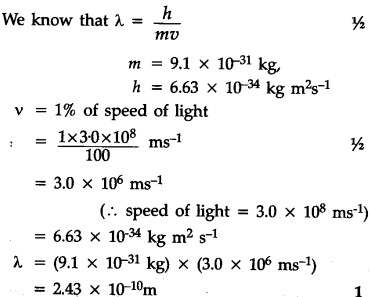# Calculate the de-Broglie wavelength of an electron (mass = 9.1 X \${{10}^{-31}}\$ kg) moving at 1% speed of light (h = 6.63 X \${{10}^{-34}}\$ kg \${{m}^{2}}\$ \${{s}^{-1}}\$